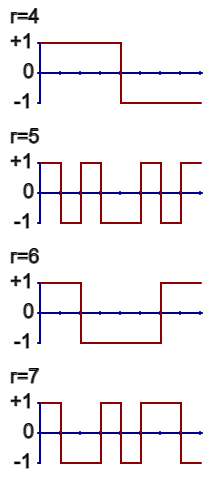# WHT: A Simpler Version of the fast Fourier Transform (FFT) you should know

The fast Walsh Hadamard transform is a simple and useful algorithm for machine learning that was popular in the 1960s and early 1970s. This useful approach should be more widely appreciated and applied for its efficiency.

By Sean O'Connor, a science and technology author and investigator.

The fast Walsh Hadamard transform (WHT) is a simplified version of the Fast Fourier Transform (FFT.)

The 2-point WHT of the sequence a, b is just the sum and difference of the 2 values:

```WHT(a, b) = a+b, a-b.

```

It is self-inverse allowing for a fixed constant:

```WHT(a+b, a-b) = 2a, 2b

```

Due to (a+b) + (a-b) = 2a and (a+b) - (a-b) = 2b.

The constant can be split between the two Walsh Hadamard transforms using a scaling factor of √2 to give a normalized WHTN:

```WHTN(a, b) = (a+b)/√2, (a-b)/√2

WHTN((a+b)/√2, (a-b)/√2) = a, b

```

That particular constant results in the vector length of a, b being unchanged after transformation since a2+b2 =((a+b)/√2)2+ ((a-b)/√2)2 as you may easily calculate.

The 2-point transform can be extended to longer sequences by sequentially adding and subtracting pairs of similar terms, alike in the pattern of + and – symbols they contain.

To transform a 4-point sequence a, b, c, d first do two 2-point transforms:

```WHT(a, b) = a+b, a-b

WHT(c, d) = c+d, c-d

```

Then add and subtract the alike terms a+b and c+d:

```WHT(a+b, c+d) = a+b+c+d, a+b-c-d

```

and the alike terms a-b and c-d:

```WHT(a-b, c-d) = a-b+c-d, a-b-c+d

```

The 4-point transform of a, b, c, d then is

```WHT(a, b, c, d) = a+b+c+d,  a+b-c-d, a-b+c-d, a-b-c+d

```

When there are no more similar terms to add and subtract, that signals completion (after log2(n) stages, where n is 4 in this case.)  The computational cost of the algorithm is nlog2(n) add/subtract operations, where n, the size of the transform, is restricted to being a positive integer power of 2 in the general case.

If the transform was done using matrix operations, the cost would be much higher (n2 fused multiply-add operations.)Figure 1.  The 4-point Walsh Hadamard transform calculated in matrix form.

The +1, -1 entries in Figure 1 are presented in a certain natural order which most of the actual algorithms for calculating the WHT result in, which is fortunate since then the matrix is symmetric, orthogonal and self-inverse.

You can also view the +1, -1 patterns of the WHT as waveforms.Figure 2.  The waveforms of the 8-point WHT presented in natural order.

When you calculate the WHT of a sequence of numbers, you are really just determining how much of each waveform is embedded in the original sequence.  And that is complete and total information with which you can fully reconstruct any sequence from its transform.

The waveforms of the WHT typically correlate strongly with the patterns found in natural data like images, allowing the transform to be used for data compression.Figure 3.  A 65536-pixel image compressed to 5000 points using a WHT.

In Figure 3, a 65536-pixel image was transformed with a WHT, the 5000 maximum magnitude embeddings were preserved, and then the inverse transform was applied (simply another WHT.)

The central limit theorem (CLT) tells you that adding a large quantity of random numbers results in the Normal distribution with its characteristic bell curve.  The CLT applies equally to sums and differences of a large quantity of random numbers.  As a result, C.M. Rader proposed (in 1969) using the WHT to quickly generate Normally distributed random numbers from conventional uniformly distributed random numbers.  You simply generate a sequence of uniform random numbers, say between –1 and 1, and then transform them using the WHT.

Similarly, you can disrupt the orderly waveform patterns of the WHT by choosing a fixed randomly chosen pattern of sign flips to apply to any input to the transform.  That is equivalent to multiplying the WHT matrix H with a diagonal matrix D of randomly chosen +1, -1 entries giving HD.  The disrupted waveform patterns in HD then fail to correlate with any of the patterns seen in natural data.  As a result, the output of HD has the Normal distribution and is actually a fast Random Projection of the natural data.  Random projections have a wide number of applications in machine learning, such as locality sensitive hashing, compressive sensing, random projection trees, neural network pre and post-processing etc.

### References

Normal Distribution:

Random Projections:

Other Applications:

Related: